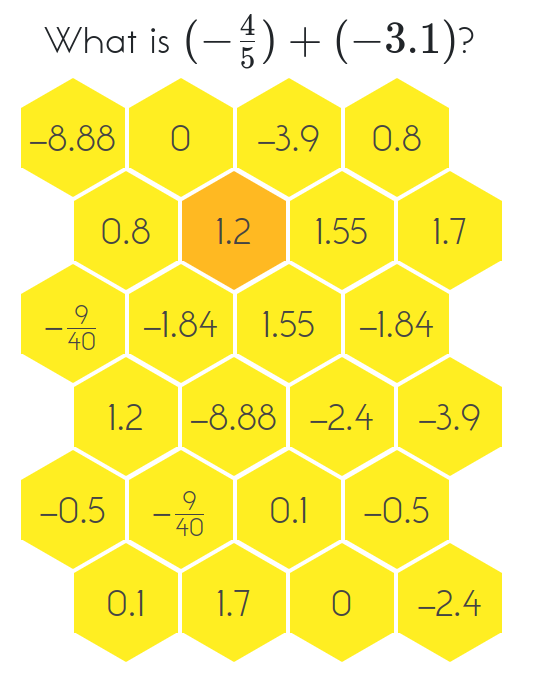MM Practice
×
Multiplication
Division
Place Value
Fractions & Decimals
Measurement
Statistics & Probability
Pre-Algebra
Money
Kindergarten
Geometry

# Hexingo - Operations with rational numbersOnline practice for grades 7-8

Practice adding, subtracting, and multiplying a mixture of fractions and decimals (some are negative) in this fun game! To win, you need to get four correct answers in a row on the hexagon-tiled board.

You can choose to include or not include three different operations: addition, subtraction, and multiplication. The game will give you math problems that always include both a fraction and a decimal, such as 1/2 + 0.2 or −0.5 − 1/5. There are also two difficulty levels to choose from.

Difficulty level:

Operations:

Screenshot: